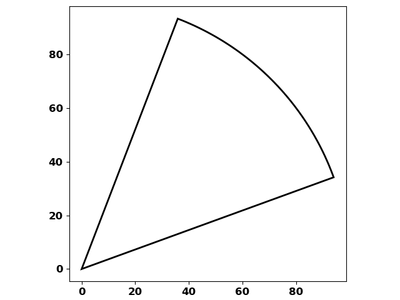# Create segment of circle based on point and attribute for radius?

444
3
04-06-2022 02:02 PMbyMVP Regular Contributor

Can anyone suggest a python snippet that could generate a segment of a circle, with the cetnre of the circle defined by a point feature class, and the radius of the segment based on an attribute from the point?

The segment angle can be hard coded, and based on a segment that subtends  northwest through to due east.

The reason for this script, is to generate indicative shadow areas for trees, with the radius based on the tree's max height value.

David
..Maps with no limits..
Tags (5)
3 RepliesbyMVP Esteemed Contributor

sector code

``````def _arc_sector(radius=100, start=0, stop=1, step=0.1, xc=0.0, yc=0.0):
"""Create an arc from a specified radius, centre and start/stop angles

Requires:
---------
cirle radius from which the arc is obtained
`start`, `stop`, `step` : numbers
angles in degrees
`xc`, `yc` : number
center coordinates in projected units

Returns:
--------
points on the arc
"""
start, stop = sorted([start, stop])
x_s = radius*np.cos(angle)         # X values
y_s = radius*np.sin(angle)         # Y values
pnts = np.c_[x_s, y_s]
cent = np.array([xc, yc])
pnts = pnts + cent
sector = np.concatenate((cent[None, :], pnts, cent[None, :]), axis=0)
return sector``````example call, it returns a numpy array.

a = _arc_sector(radius=100, start=20, stop=70, step=1, xc=0.0, yc=0.0)

angle from 20 to 70 (relative to N over 0 to 360) in 1 degree steps with center xc, yc)

If you need a list

a_list = a.tolist()

... sort of retired...byMVP Regular Contributor

@DanPatterson great thank you. So if it generates a numpy array of points, i can use the following to generate a polygon:

``````a_list = array.tolist()

# Create a feature class with a spatial reference of OSGB1936
result = arcpy.management.CreateFeatureclass(r'<GDBLocn>',
feature_class = result

# Write feature to new feature class
with arcpy.da.InsertCursor(feature_class, ['SHAPE@']) as cursor:
cursor.insertRow([a_list])``````

worked a treat. you're a star 🌟

David
..Maps with no limits..byMVP Esteemed Contributor